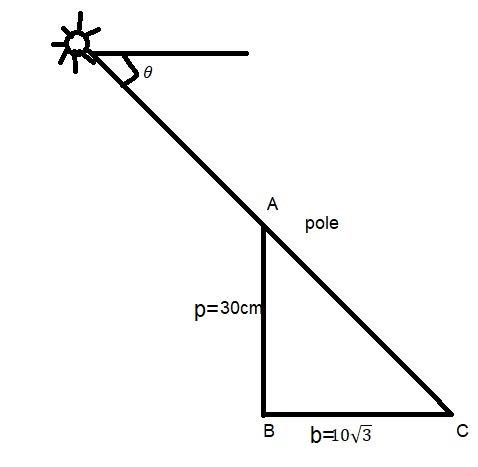Courses
Courses for Kids
Free study material
Free LIVE classes
More# Find the angular elevation (angle of elevation from the ground level) of the sunWhen the length of the shadow of a $30cm$ long pole is $10\sqrt 3 m$.Verified
328.2k+ views
Hint: When you get a question of height and distance the best way of solving is to draw a suitable diagram of the question. And then apply trigonometric ratio for further solution. The term angle of elevation denotes the angle from the horizontal upward to an object on the observer's line of sight would be above the horizontal.${\text{AB = }}$ pole ${\text{BC = }}$ shadow ,$\angle \theta =$ angle of elevation from the ground level.
In $\Delta ABC$,
$\tan c = \frac{p}{b} = \frac{{30}}{{10\sqrt 3 }} = \sqrt 3 = \tan {60^0}$
$\angle c = {60^0} = \angle \theta$ (from alternate interior angles)